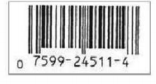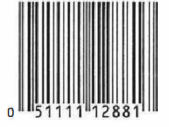Chapter 2.7, Problem 14E

Chapter
Section
Textbook Problem

Compute the check digit for the UPC symbols whose first 11 digits are given.a.b.c.d.(a)

To determine

The check digit for the UPC symbol whose first 11 digits are given.

Explanation

Given information:

Formula used:

The dot product (x1,x2,...,xn)(y1,y2,...,yn) of two ordered n-tuples(vectors) (x1,x2,...,xn) and (y1,y2,...,yn) is computed by (x1,x2,...,xn)(y1,y2,...,yn)=x1y1+x2y2+...+xnyn.

Explanation:

UPC symbol consists of 12 digits, x1x2x3x4x5x6x7x8x9x10x11x12, with last, x12, being the check digit.

The weighting vector used for the UPC symbols is the 11 – tuple (3,1,3,1,3,1,3,1,3,1,3).

The check digit x12 can be computed as,

(x1,x2,x3,x4,x5,x6,x7,x8,x9,x10,x11)(3,1,3,1,3,1,3,1,3,1,3)x12(mod10)

Here, the 11 digit code is x1x2x3x4x5x6x7x8x9x10x11=07599245114

The computation

(0,7,5,9,

(b)

To determine

The check digit for the UPC symbol whose first 11 digits are given.

(c)

To determine

The check digit for the UPC symbol whose first 11 digits are given.

(d)

To determine

The check digit for the UPC symbol whose first 11 digits are given.

Still sussing out bartleby?

Check out a sample textbook solution.

See a sample solution

The Solution to Your Study Problems

Bartleby provides explanations to thousands of textbook problems written by our experts, many with advanced degrees!

Get Started

Differentiate. f(x)=xx+cx

Single Variable Calculus: Early Transcendentals, Volume I

In Exercises 5-8, solve for x. 3x2x224x=0

Calculus: An Applied Approach (MindTap Course List)

Sometimes, Always, or Never: fxy = fyx.

Study Guide for Stewart's Multivariable Calculus, 8th

ex(ex)2 = a) ex3 b) e3x c) 3ex d) e4x

Study Guide for Stewart's Single Variable Calculus: Early Transcendentals, 8th